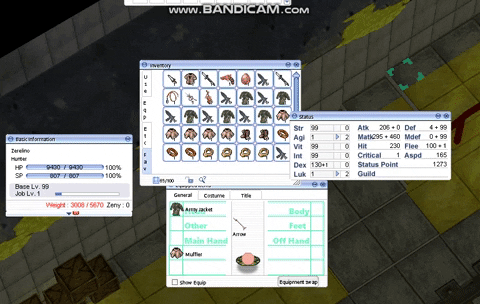## Recommended Posts

Working on my UI and didnt find a practical use for garment slot and a few personal changes , but its a little more complicated to remove and ill save it for the second postso as you see im equiping into the old shoe slot, and once all my slots are filled the shoe slot gets replaced

pc.h

find

`#define EQP_ACC (EQP_ACC_L|EQP_ACC_R)`

replace with

`#define EQP_ACC (EQP_ACC_L|EQP_ACC_R|EQP_SHOES)`

pc.c

find this, top of a block of code

```if(pos == EQP_ACC) {
pos = req_pos&EQP_ACC;
if (pos == EQP_ACC)
pos = sd->equip_index[EQI_ACC_R] >= 0 ? EQP_ACC_L : EQP_ACC_R;
}```

replace it with this

```	if(pos == EQP_ACC) {
pos = req_pos&EQP_ACC;
if (pos == EQP_ACC && (sd->equip_index[EQI_ACC_R] >= 1))
pos = sd->equip_index[EQI_ACC_L] >= 0 ? EQP_SHOES : EQP_ACC_L;
else if(pos == EQP_ACC)
pos = sd->equip_index[EQI_ACC_R] >= 0 ? EQP_ACC_L : EQP_ACC_R;
}```

Couldnt figure out what the

pos = req_pos&eqp_acc .... does but whatever

so basically this part is in charge of where the first accessory is place ( on your right looking straight at your equipment window )

`			pos = sd->equip_index[EQI_ACC_R] >= 0 ? EQP_ACC_L : EQP_ACC_R;`

and if false, it picks the second slot and replaces it

so my addition, takes highest priority, changing the entire structure * * * *

```		if (pos == EQP_ACC && (sd->equip_index[EQI_ACC_R] >= 1))
pos = sd->equip_index[EQI_ACC_L] >= 0 ? EQP_SHOES : EQP_ACC_L;```

so now we check if the right accessory is filled and if it is, continue with this line

now we replace shoe slot instead of right accessory##### Share on other sites

cant stop my client from blowing up doing it with garment, but older clients can probably get away with it, all your have to do is change the structure of it again and add the new one on top

```	if(pos == EQP_ACC) {
pos = req_pos&EQP_ACC;
if (pos == EQP_ACC && (sd->equip_index[EQP_ACC_L] >= 1))
pos = sd->equip_index[EQP_SHOES] >= 0 ? EQP_GARMENT : EQP_SHOES;

else if (pos == EQP_ACC && (sd->equip_index[EQI_ACC_R] >= 1))
pos = sd->equip_index[EQI_ACC_L] >= 0 ? EQP_SHOES : EQP_ACC_L;

else if(pos == EQP_ACC)
pos = sd->equip_index[EQI_ACC_R] >= 0 ? EQP_ACC_L : EQP_ACC_R;

}```

and again in the pc.h just add EQP_GARMENT to eqp_acc

Edited by Naruto

## Join the conversation

You can post now and register later. If you have an account, sign in now to post with your account.Reply to this topic...

×   Pasted as rich text.   Paste as plain text instead

Only 75 emoji are allowed.